info@biomedres.us   +1 (720) 414-3554
One Westbrook Corporate Center, Suite 300, Westchester, IL 60154, USA

# Biomedical Journal of Scientific & Technical Research

September, 2019, Volume 22, 4, pp 16878-16880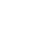Short Communication

Short Communication

# Multilayer Actuator for Nano Biomedicine

### Afonin SM*

Author Affiliations

National Research University of Electronic Technology, Russia

Received: November 06, 2019 | Published: November 12, 2019

Corresponding author: Afonin SM, National Research University of Electronic Technology, MIET, Moscow, Russia

DOI: 10.26717/BJSTR.2019.22.003795

#### Abstract

In this work we determined the mathematical description of multilayer actuator for nano biomedicine. The displacements of the multilayer actuator are received from its mathematical description.

Keywords: Multilayer actuator; Mathematical description

#### Introduction

For mathematical description of the multilayer actuator we used the equation of the relative deformation, the mechanical fourterminal scheme and the boundary conditions [1-30].

#### Mathematical Description Actuator

The equation Si relative deformation [7-11] has the following formwhere mi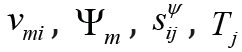are parameters

We received the equation the causes force in the formwhere S0 is the area actuator.

For the mechanical four-terminal scheme  actuator we have the matrix in the form.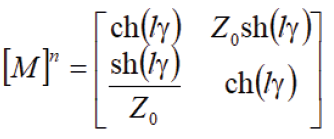where l, γ are length and coefficient.

The mathematical description and diagram on Figure 1 of the multilayer actuator we obtained as the system of the equations in the form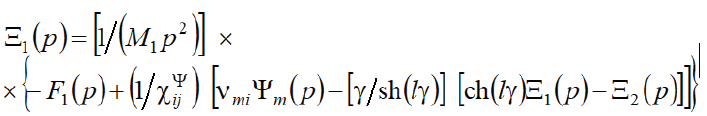whereare the Laplace transforms of the displacements and forces for the faces.

From the mathematical description of the multilayer actuator we have the matrix equation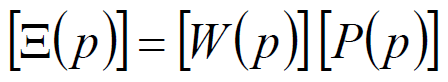where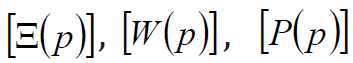are the matrices.

For time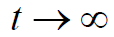for the inertial load on the two faces of piezoactuator we obtain the expressions of its displacements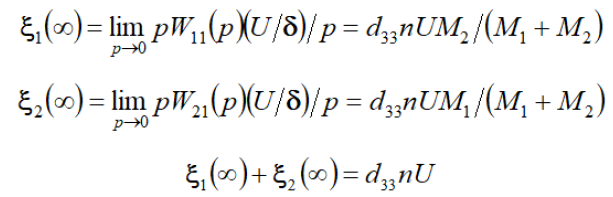where n, U are the number piezolayers and the voltage.

Atkg we obtained the displacements= 160 nm.

#### Conclusion

We determined the mathematical description of the multilayer actuator for nano biomedicine. We obtained the displacements of the multilayer actuator from its mathematical description.

#### References

Short Communication

# Multilayer Actuator for Nano Biomedicine

### Afonin SM*

Author Affiliations

National Research University of Electronic Technology, Russia

Received: November 06, 2019 | Published: November 12, 2019

Corresponding author: Afonin SM, National Research University of Electronic Technology, MIET, Moscow, Russia

DOI: 10.26717/BJSTR.2019.22.003795

#### Abstract

In this work we determined the mathematical description of multilayer actuator for nano biomedicine. The displacements of the multilayer actuator are received from its mathematical description.

Keywords: Multilayer actuator; Mathematical description